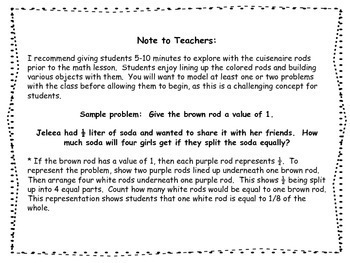# Cuisenaire Rod Bundle! Multiplying and Dividing with FractionsSubject
Resource Type
File Type

Zip

(5 MB|27 pages)
Product Rating
Standards
3 Products in this Bundle
3 products
1. Cuisenaire rods are the perfect way for students to gain an in-depth understanding of dividing fractions by whole numbers. This hands-on activity includes 16 task cards. 8 of the task cards require the use of cuisenaire rods, 4 cards require students to match an expression to a word problem, and 4
2. Cuisenaire rods are the perfect way for students to gain an in-depth understanding of dividing whole numbers by fractions. This hands-on activity includes 14 task cards (1 is a challenge card), a recording sheet, and an answer key. Each task card assigns a value to a specific rod and states a word p
3. Cuisenaire rods are the perfect way for students to gain an in-depth understanding of multiplying mixed numbers by whole numbers. This hands-on activity includes 16 task cards (3 are challenge cards), a recording sheet, and an answer key. Each task card assigns a value to a specific colored rod an
• Bundle Description
• StandardsNEW

NEW BUNDLE! Multiplying and dividing with fractions are difficult for students to conceptualize! Using Cuisenaire rods is a great hands-on approach to help students truly understand the concept. This bundle includes 3 resources: Dividing Fractions by Whole Numbers, Dividing Whole Numbers by Fractions, and Multiplying Mixed Numbers by Whole Numbers. Each resource includes task cards (requiring the use of Cuisenaire rods), an explanation with sample problems, a recording sheet, and answer key. Best of all... students have FUN when learning with Cuisenaire rods!

Apply and extend previous understandings of division to divide unit fractions by whole numbers and whole numbers by unit fractions.
Solve real world problems involving multiplication of fractions and mixed numbers, e.g., by using visual fraction models or equations to represent the problem.
Interpret multiplication as scaling (resizing), by:
Apply and extend previous understandings of multiplication to multiply a fraction or whole number by a fraction.
Total Pages
27 pages
Included
Teaching Duration
N/A
Report this Resource to TpT
Reported resources will be reviewed by our team. Report this resource to let us know if this resource violates TpT’s content guidelines.